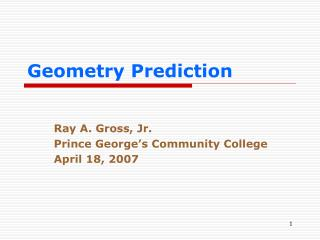DownloadDownload PresentationGeometry Prediction

# Geometry Prediction

Télécharger la présentation## Geometry Prediction

- - - - - - - - - - - - - - - - - - - - - - - - - - - E N D - - - - - - - - - - - - - - - - - - - - - - - - - - -
##### Presentation Transcript

1. Geometry Prediction Ray A. Gross, Jr. Prince George’s Community College April 18, 2007

2. Nature of Bonding • Central Atom (CA) • Ligands (L) • Lone Pairs (LPCA)

3. G. N. LewisLinus Pauling 1930 1931

4. Water (H2O)

5. Sulfuric Acid (H2SO4) Note: L = # s bonds to CA.

6. Nature of Bonding • Hybrid orbitals (HO) • p (unhybridized)orbitals HO = L+ LPCA

7. Water HO = L + LPCA= 2 + 2 = 4

8. Sulfuric Acid HO = L + LPCA = 4 + 0 = 4

9. Hybridization tells us the number of hybrid orbitals (HO) • sp = two hybrid orbitals, or HO = 2 • sp3 = three hybrid orbitals, or HO = 3 • sp3d2= six hybrid orbitals, or HO = 6 If we know the hybridization, we know HO. If we know HO, we know the hybridization.

10. Geometry determined by any two of HO, L and LPCA Water Example

11. Geometry determined by any two of HO, L and LPCA Sulfuric Acid Example

12. Gross Procedure: Determine Hybridization and Geometry by finding L and LPCA • Find L and LPCA • L + LPCA = HO • HO hybridization • HO and L  geometry

13. Example: BH3 • Find L; L = 3 • Find LPCA; LPCA = ?

14. Example: BH3 LPCA = half the CA’s electrons leftover after bonding.

15. LPCA = half the CA’s electrons leftover after bonding. • Let ERL = CA’s electrons required by L • Let VE = CA’s valence electrons Then LPCA = ½(VE – ERL)

16. LPCA = ½(VE – ERL) • For BH3: VE = 3, ERL = 3 (1 per H) LPCA = ½(3 – 3) = 0

17. Example: BH3 • Find L; L = 3 • Find LPCA; LPCA = 0 • Thus, • HO = 3; hybridization = sp2 • L = 3; geometry = trigonal planar

18. Example: NH3 Each H requires one of CA’s electrons.

19. Example: NH3 • L = 3 • LPCA = ½(VE – ERL) = ½(5 – 3) = 1 • HO = L + LPCA = 3 + 1 = 4 = sp3 • HO = 4, L = 3 = trigonal pyramidal

20. Example: H2SO4 Each O requires two electrons; each OH requires one electron.

21. Example: H2SO4 = (HO)2SO2 • L = 4 • LPCA = ½(VE – ERL) • LPCA = ½[6 – (2 x 1) – (2 x 2) = 0 • HO = 4, L = 4 • sp3; tetrahedral

22. Example: GeCl4-2 • VE = 4 + 2 = 6 • ERL = 4 x 1 = 4 • LPCA = ½(6 – 4) = 1 • L = 4 • HO = L + LPCA = 5 • HO = 5, L = 4 • sp3d, seesaw geometry

23. Findings Available electrons, VEadj= VE adjusted for charge CH • VEadj = VE – CH Electrons Required by Ligands (ERL) • ERL = Normal Covalence for atoms • ERL = # for L atom next to CA to complete its octet. H and O as ligands • H and O peripheral atoms equal an OH unitary ligand.

24. Five-step Procedure

25. What is the geometry of HNO3? • N = CA; VEadj= 5 – 0 = 5 • L = OH and 2 O’s = 3 • ERL = 1 + 2 x 2 = 5 • LPCA = ½(5 – 5) = 0 • HO = 3; L = 3 • sp2; trigonal planar

26. Demo GP

27. Organic Procedure

28. Demo GP

29. Conclusions • GP combines inorganic and organic procedures. • GP gives fast, accurate results.

30. Acknowledgements • Thanks to all who came to my last seminar. • I was inspired by it to do this work. RAG